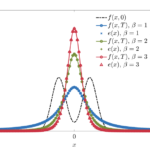# On a class of Fokker–Planck equations with subcritical confinementG. Toscani, M. Zanella

Atti Accad. Naz. Lincei Rend. Lincei Mat. Appl., 32:471-496, 2021. (Preprint arXiv)

We study the relaxation to equilibrium for a class linear one-dimensional Fokker–Planck equations characterized by a particular subcritical confinement potential.
An interesting feature of this class of Fokker–Planck equations is that, for any given probability density \$e(x)\$, the diffusion coefficient can be built to have \$e(x)\$ as steady state. This representation of the equilibrium density can be fruitfully used to obtain one-dimensional Wirtinger-type inequalities and to recover, for a sufficiently regular density \$e(x) \$, a polynomial rate of convergence to equilibrium.Numerical results then confirm the theoretical analysis, and allow to conjecture that convergence to equilibrium with positive rate still holds for steady states characterized by a very slow polynomial decay at infinity.